# Yes the STEELERS LOST yesterday Graphs of Polynomial

• Slides: 21Yes, the STEELERS LOST yesterday!Graphs of Polynomial Functions E. Q: What can we learn about a polynomial from its graph?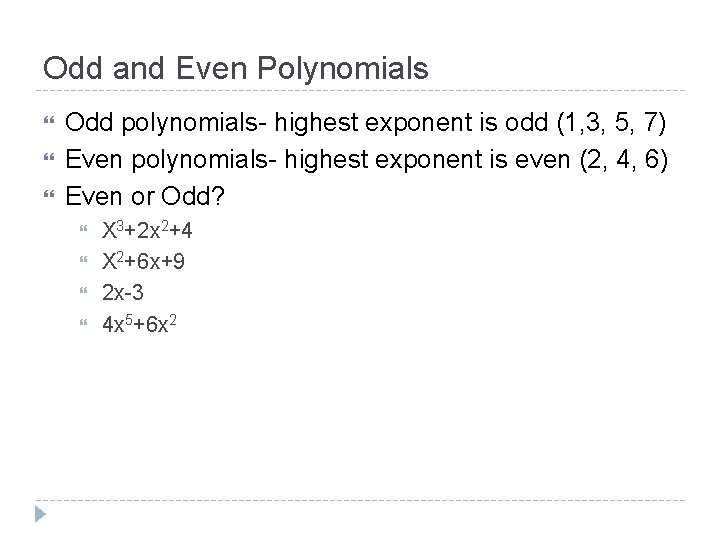Odd and Even Polynomials Odd polynomials- highest exponent is odd (1, 3, 5, 7) Even polynomials- highest exponent is even (2, 4, 6) Even or Odd? X 3+2 x 2+4 X 2+6 x+9 2 x-3 4 x 5+6 x 2Odd Polynomials One end of the graph falls One end of the graph rises Does not matter what is in betweenEnd Behavior The end behavior of the graph of the polynomial is the same as the end behavior of the graph of the leading term or highest exponent. Look at the very ends of the graph to determine Odd polynomials: One side of the graph rises, and one side of the graph falls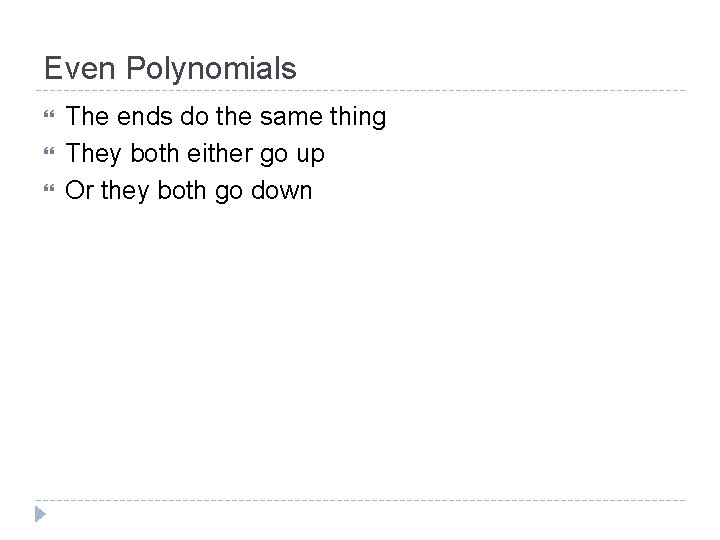Even Polynomials The ends do the same thing They both either go up Or they both go downEnd Behavior Even polynomials: Both ends rise, and both ends fallLeading Coefficient The number in front of the highest exponent is the leading coefficient The number itself does not matter Need to determine if it is positive or negative Changes the end behavior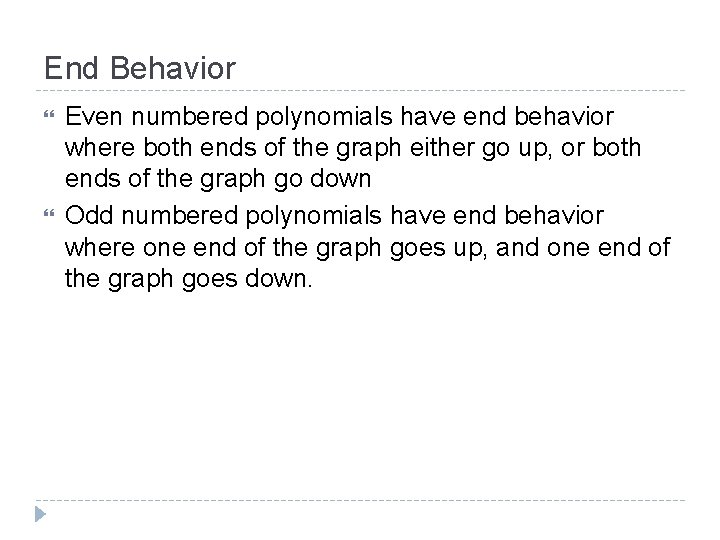End Behavior Even numbered polynomials have end behavior where both ends of the graph either go up, or both ends of the graph go down Odd numbered polynomials have end behavior where one end of the graph goes up, and one end of the graph goes down.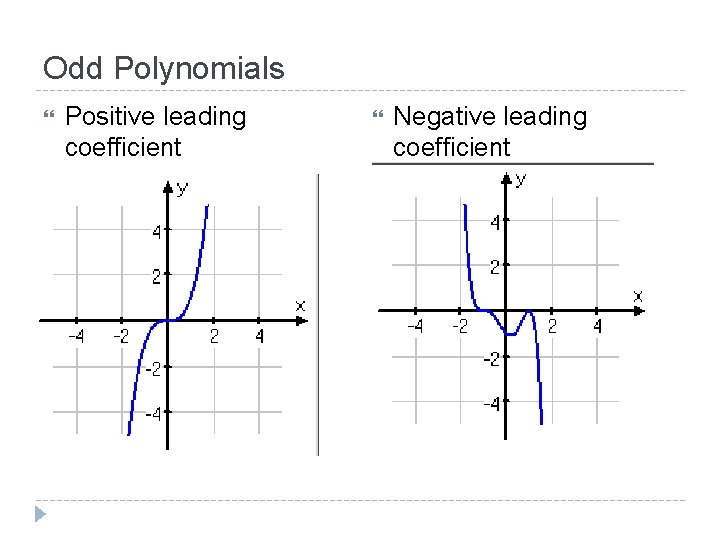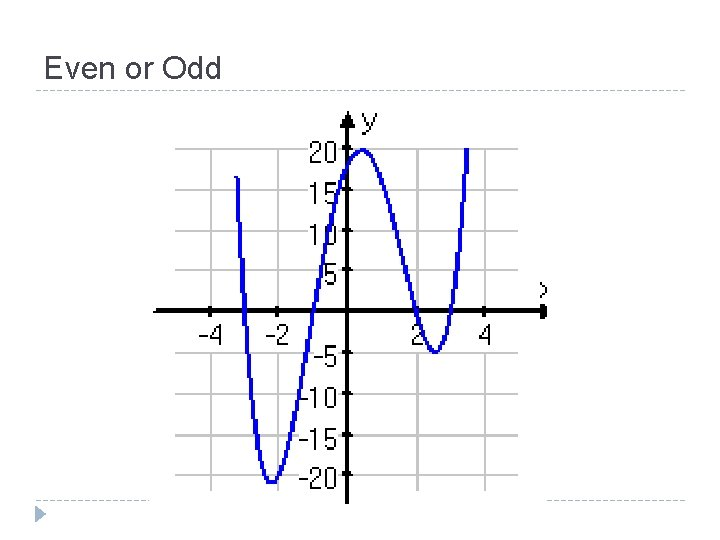Even or Odd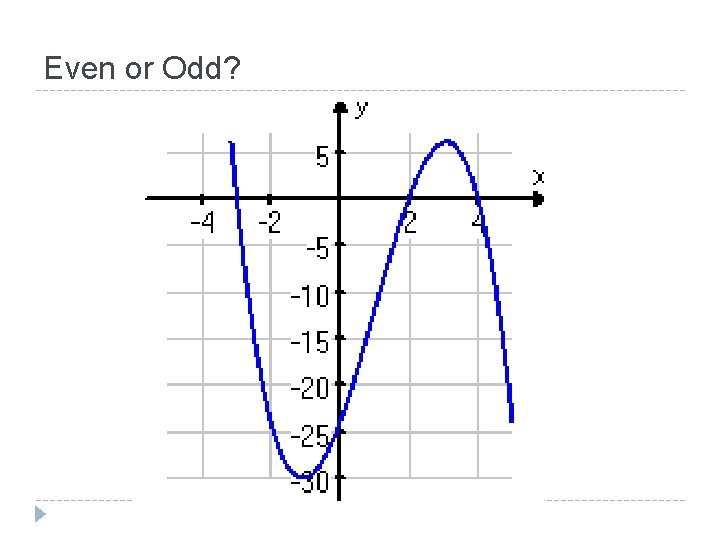Even or Odd?Describe the end behavior of 3 x 7+5 x+1040Intercepts For any polynomial function Y intercept is the constant term in the equation (the one without the x attached) X intercepts are the real zeros of the polynomial (we will use the calculator to find them) A polynomial will always have one y intercept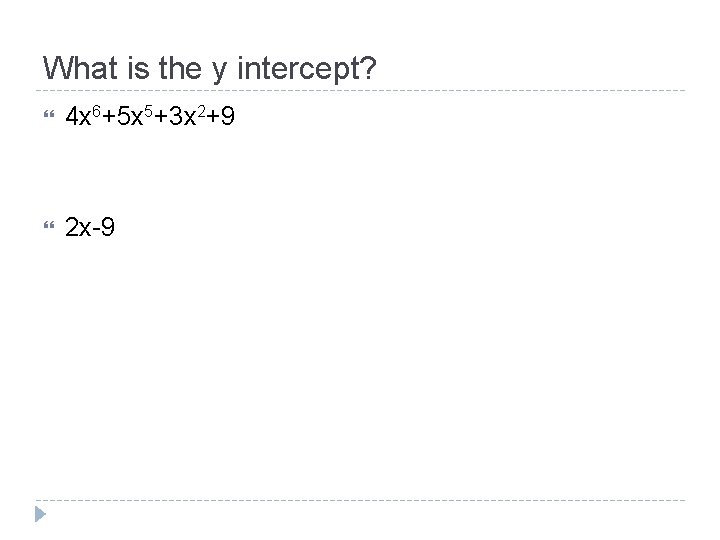What is the y intercept? 4 x 6+5 x 5+3 x 2+9 2 x-9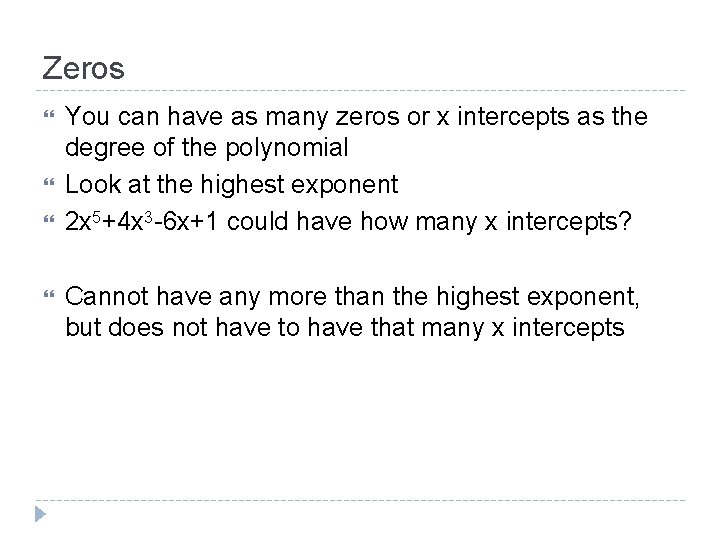Zeros You can have as many zeros or x intercepts as the degree of the polynomial Look at the highest exponent 2 x 5+4 x 3 -6 x+1 could have how many x intercepts? Cannot have any more than the highest exponent, but does not have to have that many x intercepts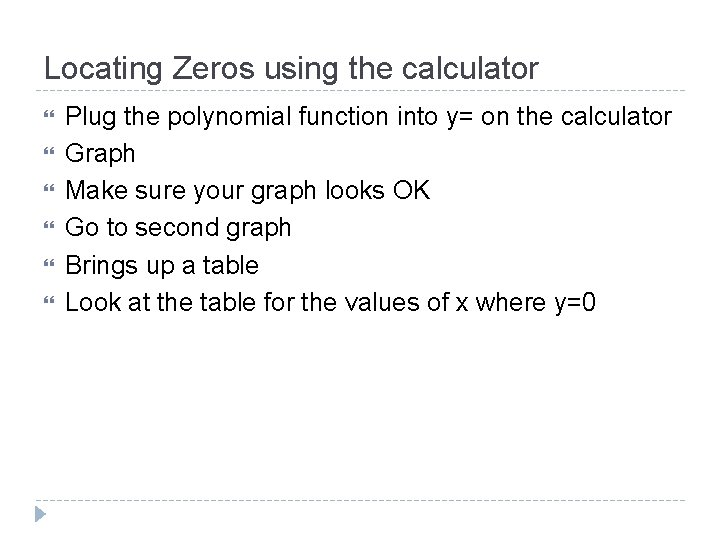Locating Zeros using the calculator Plug the polynomial function into y= on the calculator Graph Make sure your graph looks OK Go to second graph Brings up a table Look at the table for the values of x where y=0Find the zeros X 3 -2 x 2 -5 x+6Find the zeros X 3+4 x 2 -x-4Find the zeros X 3+4 x 2 -x-4